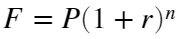# Problem 56120. Compound Interest : Compounding Periods

Theorem : Compound Interestwhere,
• F : Future value at the end of n periods
• P : Present value
• r : Annual nominal rate
• n : Total number of compounding periods
Calculate n, given F, P and r.

### Solution Stats

79.31% Correct | 20.69% Incorrect
Last Solution submitted on Oct 06, 2023

### Community Treasure Hunt

Find the treasures in MATLAB Central and discover how the community can help you!

Start Hunting!